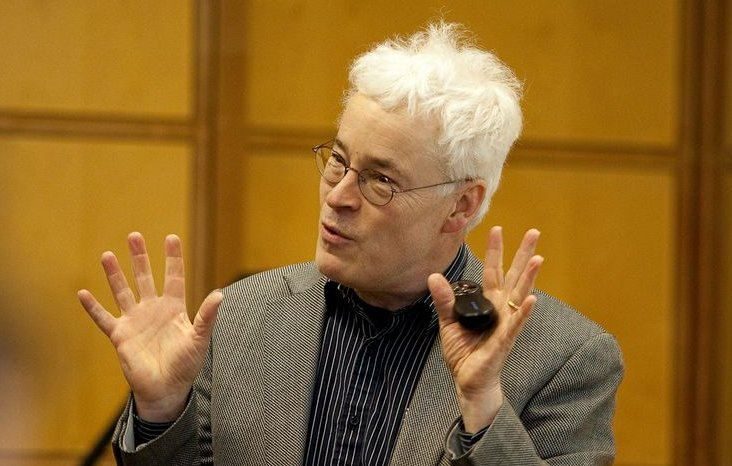## Trefethen's NYU Lectures on Approximation Theory and Approximation PracticePhoto by Sara Kerens

In Sept-Oct 2015 Nick Trefethen, Professor of Numerical Analysis at Oxford and Global Distinguished Professor at NYU, gave an 18-hour series of lectures at NYU presenting all 28 chapters of this book. The lectures are full of unusual mathematical, philosophical, and historical perspectives, and everything is illustrated by Chebfun demos on the computer. This is a unique resource for those who want a deeper understanding of the foundations of numerical computation.

An outline of the lectures is below. Click here for a detailed outline and here for the videos, which can be viewed at speeds 0.5x, 1x, 1.4x, 1.6x, or 2x.

```LECTURE 1
Chapter 1. Introduction [4:40]
Chapter 2. Chebyshev points and interpolants [22:00]
Chapter 3. Chebyshev polynomials and series [45:20]

LECTURE 2
Chapter 4. Interpolants, truncations, and aliasing [7:30]
Chapter 5. Barycentric interpolation formula [44:30]

LECTURE 3
Chapter 6. Weierstrass approximation theorem [0:00]
Chapter 7. Convergence for differentiable functions [39:30]

LECTURE 4
Chapter 8. Convergence for analytic functions [0:00]
Chapter 9. Gibbs phenomenon [29:40]
Chapter 10. Best approximation [46:30]

LECTURE 5
Chapter 11. Hermite integral formula [9:00]
Chapter 13. Equispaced points, Runge phenomenon [57:40]
Chapter 12. Potential theory [1:06:10]

LECTURE 6
Chapter 14. Discussion of higher-order polynomial interpolation [0:00]
Chapter 15. Lebesgue constants [17:00]

LECTURE 7
Chapter 16. Best and near-best [3:30]
Chapter 17. Orthogonal polynomials [6:50]
Chapter 18. Polynomial roots and colleague matrices [44:30]

LECTURE 8
Chapter 19. Clenshaw-Curtis and Gauss quadrature [5:30]

LECTURE 9
Chapter 20. Caratheodory-Fejer approximation [0:00]
Chapter 21. Spectral methods [40:00]

LECTURE 10
Chapter 22. Linear approximations: beyond polynomials [4:00]
Chapter 23. Nonlinear approximations: why rational functions? [24:30]
Chapter 24. Rational best approximation [1:09:20]

LECTURE 11
Chapter 25. Two famous problems [0:00]
Chapter 26. Rational interpolation and linearized least-squares [41:40]

LECTURE 12